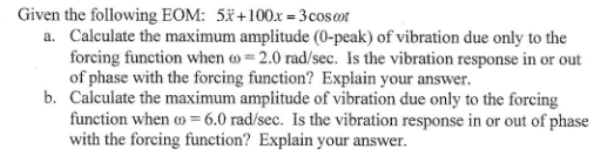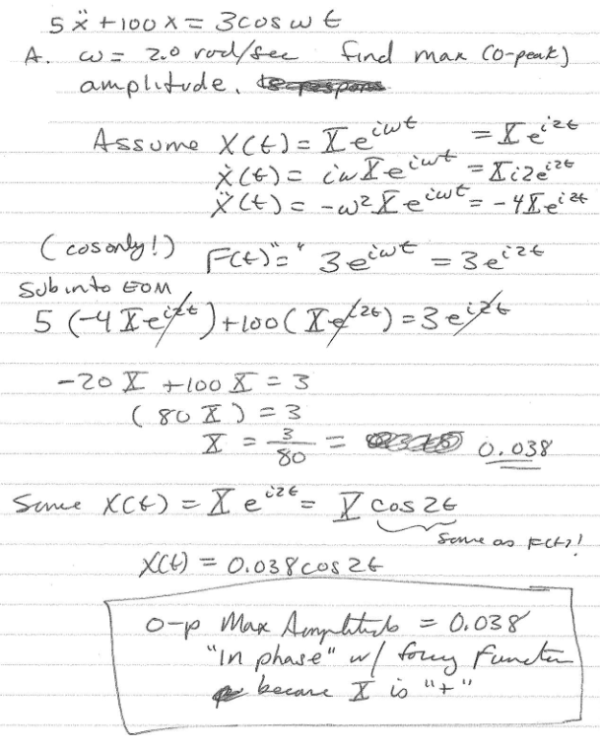Given the following EOM: 5xdoubdot + 100x=3coswt a) Calculate the maximum amplitude (0-peak) of vibration due only to the forcing function when w=2.0 rad/sec. Is the vibration response in or out of phase with the forcing function? Explain your answer. b) Calculate the maximum amplitude of vibration due only to the forcin function when w=6.0 rad/sec. Is the vibration response in or out of phase with the forcing function? Explain your answer.Given the following EOM: 5xdoubdot + 100x=3coswt a) Calculate the maximum amplitude (0-peak) of vibration due only to the forcing function when w=2.0 rad/sec. Is the vibration response in or out of phase with the forcing function? Explain your answer. b) Calculate the maximum amplitude of vibration due only to the forcin function when w=6.0 rad/sec. Is the vibration response in or out of phase with the forcing function? Explain your answer.

Vibrations Page 1 vibrations vibrations vibrations vibrations vibrations vibrations vibrations Vibrations Page 2